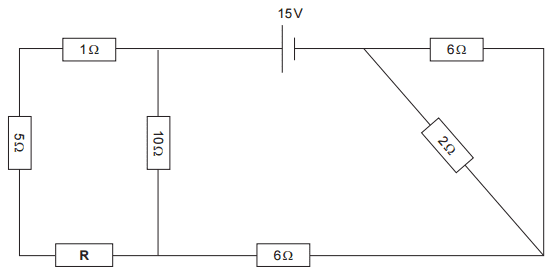# Resistance with resistors between parallel resistors

## Homework Statement

The overall resistance of the circuit is 12.5Ω. Calculate the value of the resistor labelled R in the circuit.## Homework Equations

1/R = 1/R1 + 1/R2
R = V/I

## The Attempt at a Solution

I understand that you use the first equation for parallel resistors and the second to calculate resistance from voltage and current. The bit I am having trouble with is the network of 4 resistors on the left hand side. The resistance on the right hand-side is 1/R = 1/2 + 1/6, so R = 1.5. So then 12.5 = 6 + 1.5 + the resistance of the left hand-side network.

I just need guidance on how to calculate it because it puzzles me as there is 2 resistors between the other 2 in parallel.

Last edited:

BvU
Homework Helper
there is 2 resistors between the other 2 in parallel.
A matter of drawing; one could also say there are three in series with one in parallel ...

cnh1995
Homework Helper
Gold Member
2 resistors between the other 2 in parallel.
Are you sure?
Hint:Current through series resistors is same.

Paul Colby
Gold Member
I find it helpful to follow the current around the circuit. Start at the battery. As the current flows where does it split? It's enough to start at the point in the circuit where you lost the bubble.

Thanks guys! Just considered the other 3 (5, 1 and R) as a series and the 10 as parallel with those. :)

•CWatters
CWatters
Homework Helper
Gold Member
The resistance on the right hand-side is 1/R = 1/2 + 1/6, so R = 1.5. So then 12.5 = 6 + 1.5 + the resistance of the left hand-side network.

Yes that's ok.

Thanks guys! Just considered the other 3 (5, 1 and R) as a series and the 10 as parallel with those. :)

Yes that's ok for the "left hand side network".

Just turn it all into an equation and solve for R